##### Basic Math & Pre-Algebra Workbook For Dummies with Online Practice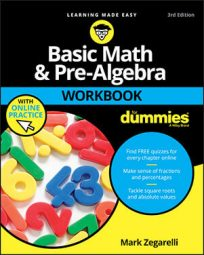Cross-multiplication is a handy tool for finding the common denominator for two fractions, which is important for many operations involving fractions. In the following practice questions, you are asked to cross-multiply to compare fractions to find out which is greater or less.

## Practice questions

1. Find the lesser fraction: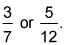2. Among these three fractions, which is greatest: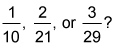1. Of the two fractions,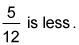Cross-multiply to compare the two fractions: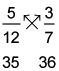Because 35 is less than 36,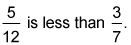2. Of the three fractions,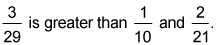Use cross-multiplication to compare the first two fractions.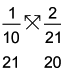Because 21 is greater than 20, this means that 1/10 is greater than 2/21, so you can rule out 2/21. Next, compare 1/10 and 3/29 by cross-multiplying.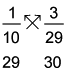Because 30 is greater than 29, 3/29 is greater than 1/10. Therefore, 3/29 is the greatest of the three fractions.